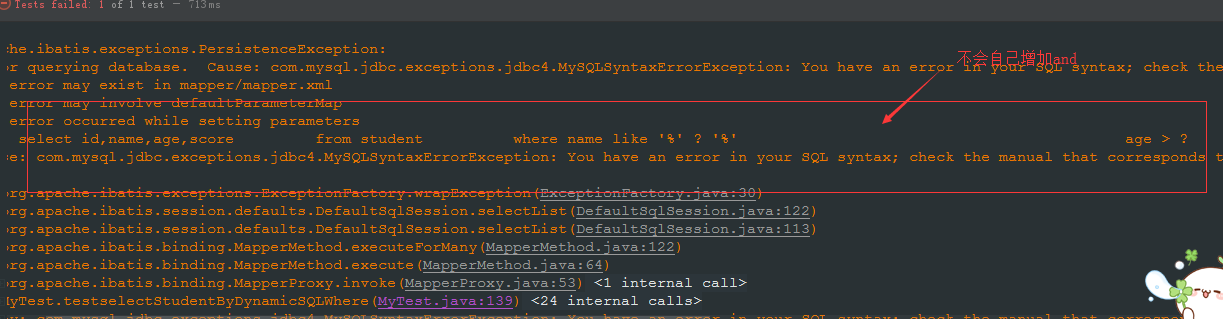# Mybatis【13】-- Mybatis 动态 Sql 标签的使用mybatis 有一个强大的特性，其他框架在拼接 sql 的时候要特别谨慎，比如哪里需要空格，还要注意去掉列表最后一个列名的逗号，mybtis 的动态 sql 可以帮助我们逃离这样的痛苦挣扎，那就是动态 SQL.它还可以处理一种情况，当你不确定你的参数不知道是不是为空的时候，我们不需要在业务逻辑中判断，直接在 sql 中处理，代码无比简洁。主要的动态 sql 标签如下：<br>

- <if></if>

- <where></where>(trim,set)

- <choose></choose>（when, otherwise）

- <foreach></foreach>

---|---|---|---|---|---|---|---

#### <if>

    <!-- 动态sql if标签-->    <!-- &可以使用and来代替 ，注意！=需要连在一起写-->    <select id="selectStudentByDynamicSQL" resultType="Student">        <!--最常用的（动态参数） select id,name,age,score from student where name like '%' #{name} '%' -->        <!-- 下面的是字符串拼接 ，只能写value，了解即可，容易sql注入，执行效率低，不建议使用-->        select id,name,age,score        from student        where 1=1        <if test="name != null and name != ''">            and name like '%' #{name} '%'        </if>        <if test="age > 0">            and age > #{age}        </if>    </select>

select * from student where 1=1<br>

<if></if>标签需要手动在 where 后面添加 1=1 语句，这是因为如果<if>后面的条件都是 false 的时候，where 后面如果没有 1=1 语句，sql 就剩下一个空空的 where,sql 就会报错。所以在 where 后面需要加上永真句子 1=1，但是这样有一个问题，当数据量比较大的时候，会严重影响 sql 的查询效率。

#### <where></where>,<trim></trim>,<set></set>

    <select id="selectStudentByDynamicSQLWhere" resultType="Student">        <!--最常用的（动态参数） select id,name,age,score from student where name like '%' #{name} '%' -->        <!-- 下面的是字符串拼接 ，只能写value，了解即可，容易sql注入，执行效率低，不建议使用-->        select id,name,age,score        from student        <where>            <if test="name != null and name != ''">                and name like '%' #{name} '%'            </if>            <if test="age > 0">                and age > #{age}            </if>        </where>    </select>

- prefix:在包含的内容前加上前缀，不是百分之百会加，会根据需要自动加

- suffix：在包含的内容后面加上后缀，不是百分之百会加，会根据需要自动加

- prefixOverrides：可以把包含内容的首部某些内容忽略（不能自己增加），不一定会忽略，根据需要自动忽略

- suffixOverrides：也可以把包含内容的尾部的某些内容忽略（不能自己增加），同上

    <select id="selectStudentByDynamicSQLWhere" resultType="Student">        select id,name,age,score        from student        <trim prefix="where" prefixOverrides="and">            <if test="name != null and name != ''">                name like '%' #{name} '%'            </if>            <if test="age > 0">                age > #{age}            </if>        </trim>    </select>

<br><br>

    <select id="selectStudentByDynamicSQLWhere" resultType="Student">        select id,name,age,score        from student        <trim prefix="where" prefixOverrides="and">            <if test="name != null and name != ''">                and name like '%' #{name} '%'            </if>            <if test="age > 0">                and age > #{age}            </if>        </trim>    </select>

update user<trim prefix="set" suffixoverride="," suffix=" where id = #{id} ">　　<if test="name != null and name.length()>0"> name=#{name} , </if>　　<if test="age != null "> age=#{age} ,  </if></trim>

    <update id="updateStudent">		update student		<set>            <!-- 第一个if标签的逗号一定要有，最后一个标签的逗号可以没有-->            <if test="name != null"> name=#{name},</if>            <if test="age != null">age=#{age},</if>            <if test="score != null"> score=#{score},</if>        </set>         where id=#{id}	</update>

#### <choose>, <when>, <otherwise>

    <!-- 	selectStudentByDynamicSQLChoose 类似于switch，满足后就不会判断后面的了-->    <!-- 如果名字不为空，那么按照名字来查询，如果名字为空，就按照年龄来查询，如果没有查询条件，就没有查询条件 -->    <select id="selectStudentByDynamicSQLChoose" resultType="Student">        <!--最常用的（动态参数） select id,name,age,score from student where name like '%' #{name} '%' -->        select id,name,age,score        from student        <where>            <choose>                <when test="name != null and name != ''">                    and name like '%' #{name} '%'                </when>                <when test="age > 0">                    and age > #{age}                </when>                <otherwise>                    and 1 != 1                </otherwise>            </choose>        </where>    </select>

<choose>标签就像是 switch 语句，每一个<when>都像是 case,后面默认跟上 break 语句，只要满足一个就不会判断后面的子语句了，当前面所有的<when></when>都不执行的时候，就会执行<otherwise></otherwise>标签的内容，这个内容也就像是 switch 语句里面的 default。

#### foreach

- collection 表示需要遍历的集合类型，array 表示需要遍历的数组

- open，close，separator 是对遍历内容的 SQL 拼接

- foreach 元素的功能非常强大，它允许你指定一个集合，声明可以在元素体内使用的集合项（item）和索引（index）变量。它也允许你指定开头与结尾的字符串以及在迭代结果之间放置分隔符。

- 你可以将任何可迭代对象（如 List、Set 等）、Map 对象或者数组对象传递给 foreach 作为集合参数。当使用可迭代对象或者数组时，index 是当前迭代的次数，item 的值是本次迭代获取的元素。当使用 Map 对象（或者 Map.Entry 对象的集合）时，index 是键，item 是值。

1.比如我们需要查找学生的 id 为 1，2，3 的学生信息，我们不希望分开一次査一个，而是希望将数组 id 一次传进去，查出来一个学生的集合。<br>

sql 接口可以这样写,传入一个对象的数组：

public List<Student>selectStudentByDynamicSQLForeachArray(Object[]studentIds);

sql 语句如下,遍历 array 数组的时候，指定左边符号是左括号，右边是右括号，元素以逗号分隔开：

    <!-- select * from student where id in (1,3) -->    <select id="selectStudentByDynamicSQLForeachArray" resultType="Student">        select id,name,age,score        from student        <if test="array !=null and array.length > 0 ">            where id in            <foreach collection="array" open="(" close=")" item="myid" separator=",">                #{myid}            </foreach>        </if>    </select>

2.当遍历的是一个类型为 int 的 list 列表时：

public List<Student>selectStudentByDynamicSQLForeachList(List<Integer>studentIds);

sql 语句如下,colleaction 指定为 list：

    <select id="selectStudentByDynamicSQLForeachList" resultType="Student">        select id,name,age,score        from student        <if test="list !=null and list.size > 0 ">            where id in            <foreach collection="list" open="(" close=")" item="myid" separator=",">                #{myid}            </foreach>        </if>    </select>

3.当遍历的是一个类型为对象的 list：

public List<Student>selectStudentByDynamicSQLForeachListStudent(List<Student>students);

sql 语句里面与上面相似，只是在使用属性的时候不太一样：

<select id="selectStudentByDynamicSQLForeachListStudent" resultType="Student">        select id,name,age,score        from student        <if test="list !=null and list.size > 0 ">            where id in            <foreach collection="list" open="(" close=")" item="stu" separator=",">                #{stu.id}            </foreach>        </if>    </select>

#### <sql></sql>

    <sql id="selectHead">		select id,name,age,score		 from student	</sql>    <!-- 可读性比较差 -->    <select id="selectStudentByDynamicSQLfragment" resultType="Student">        <include refid="selectHead"></include>        <if test="list !=null and list.size > 0 ">            where id in            <foreach collection="list" open="(" close=")" item="stu" separator=",">                #{stu.id}            </foreach>        </if>    </select>

【作者简介】## 评论